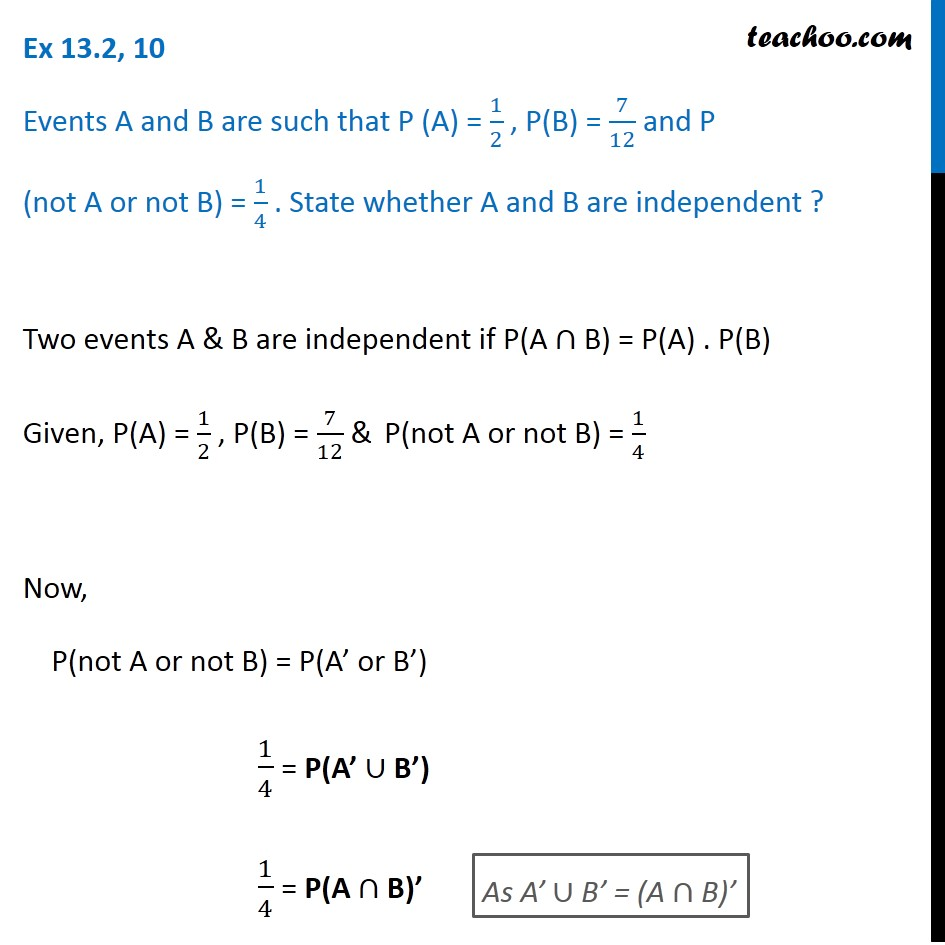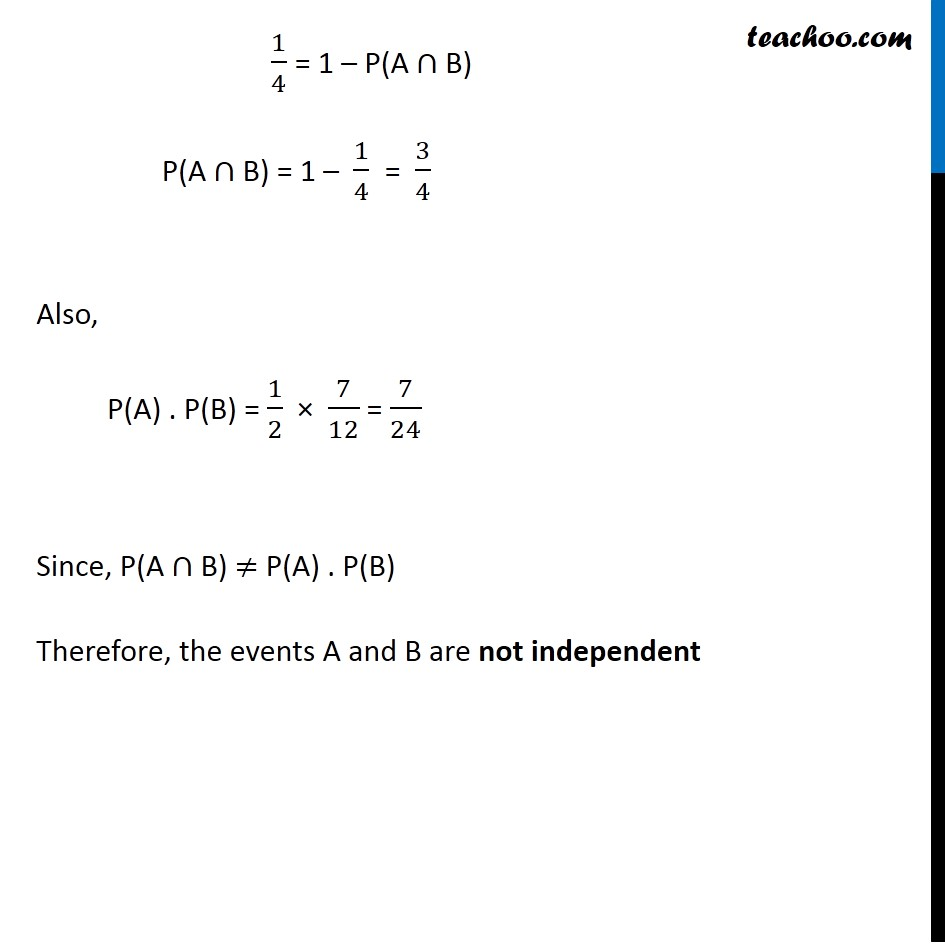Ex 13.2

Chapter 13 Class 12 Probability
Serial order wiseLearn in your speed, with individual attention - Teachoo Maths 1-on-1 Class

### Transcript

Ex 13.2, 10 Events A and B are such that P (A) = 1/2 , P(B) = 7/12 and P (not A or not B) = 1/4 . State whether A and B are independent ?Given, P(A) = 1/2 , P(B) = 7/12 & P(not A or not B) = 1/4 Now, P(not A or not B) = P(A’ or B’) 1/4 = P(A’ ∪ B’) 1/4 = P(A ∩ B)’ 1/4 = 1 – P(A ∩ B) P(A ∩ B) = 1 – 1/4 P(A ∩ B) = 3/4 Also, P(A) . P(B) = 1/2 × 7/12 = 7/24 ≠ P(A ∩ B) Since, P(A ∩ B) ≠ P(A) . P(B) Therefore, the events A and B are not independent